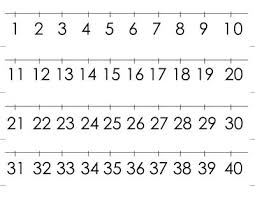# Significant figures

How many significant figures are present in each of the measured quantities?
A. 0.0012
B. 900.0
C. 108
D. .0012
E. 2006
F. 0.002070

A =  2
B =  1
C =  3
D =  2
E =  4
F =  3

### Step-by-step explanation:Did you find an error or inaccuracy? Feel free to write us. Thank you!

Showing 1 comment:
Matematik
Significant figures are the digits of a number that are meaningful in terms of accuracy or precision. They include:
A) Any non-zero digit
B) Zeros between non-zero digits as in 2003 or 56.70001
C) Trailing zeros only when there is a decimal point, as in 1730. or 935.6600

Tips for related online calculators
Need help calculating sum, simplifying, or multiplying fractions? Try our fraction calculator.